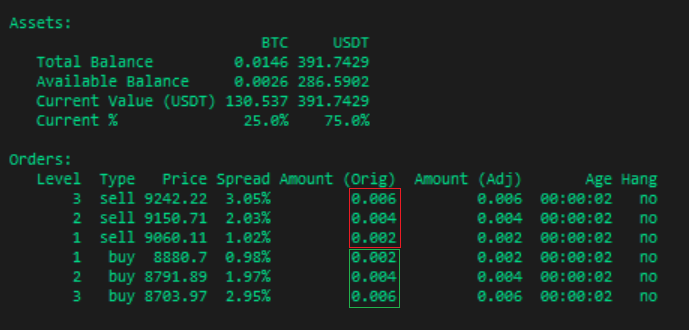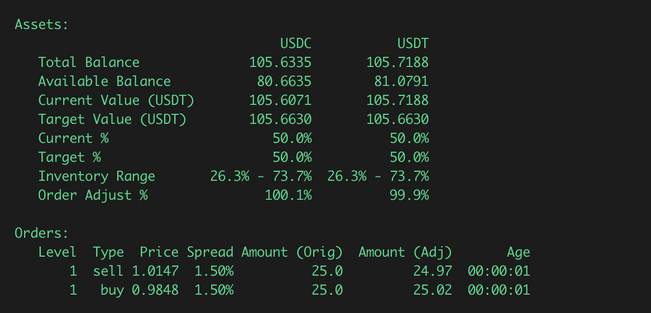# Inventory Skew¶

Updated as of v0.29.0

This feature lets you set and maintain a target inventory split between the base and quote assets. It prevents your overall inventory level from changing too much and may result in more stable performance in volatile markets.

## inventory_skew_enabled¶

Allows the user to set and maintain a target inventory split between base and quote assets.

Prompt:

Would you like to enable inventory skew? (Yes/No)
>>> Yes


## inventory_target_base_pct¶

Target amount held of the base asset, expressed as a percentage of the total base and quote asset value.

Prompt:

On [exchange], you have [base_asset_balance] and [quote_asset_balance]. By market value, your current inventory split is [base_%_ratio] and [quote_%_ratio]. Would you like to keep this ratio?
>>> Yes


## inventory_range_multiplier¶

This expands the range of tolerable inventory level around your target base percent, as a multiple of your total order size. Larger values expand this range.

Prompt:

What is your tolerable range of inventory around the target, expressed in multiples of your total order size?
>>>


## How it works¶

This function adjusts the bid and ask order amounts to limit the user's trading exposure within a defined range. This prevents the user from being over-exposed from the risks of a single side of the trade when the market keeps hitting limit orders on one side only.

Note

Example: You are market making for the BTC-USDT pair and have 0.667 BTC and 6000 USDT. At $6000 BTC price, your total portfolio value is$10,000 and the base asset (BTC) accounts for 40% of total value. If your target base percent is 50%, your buy orders will be increased and your sell orders will be decreased until you reach the target percent.

The user specifies a target base asset percentage. Since the user's outstanding orders may change this split if they are filled, the total order size is used to define an allowable range around this target percentage. The user may expand or contract this range via a multiplier parameter.

Note

Example: You are market making for the BTC-USDT pair and the total value of your BTC/USDT inventory is 10 BTC. Your target base percent is 50% and each set of orders you place is 1 BTC (10% of your total portfolio). With inventory_range_multiplier of 1.00, your target range is 40% to 60%. With inventory_range_multiplier of 2.00, your target range is 30% to 70%.

If the user's base asset value goes above the upper limit, then no bid orders would be emitted. Conversely, if the user's base asset value goes below the lower limit, then no ask orders would be emitted.

## Sample configurations¶

- market: BTC-USDT
- order_amount: 0.002
- order_levels: 3
- order_level_amount: 0.002


### No inventory skew¶

- inventory_skew_enabled: FalseWithout inventory skew, order amounts are always symmetrical between buy (outlined in green) and sell orders (outlined in red).

### Inventory skew, multiplier = 1¶

- inventory_skew_enabled: True
- inventory_target_base_pct: 50
- inventory_range_multiplier: 1.0Since the current inventory range of each asset is within the target range (8.7% - 91.3%), both buy and sell orders are placed. However, more buy orders will be created with larger order amounts than the sell order amounts.

### Inventory skew, multiplier = 0.5¶

- inventory_skew_enabled: True
- inventory_target_base_pct: 50
- inventory_range_multiplier: 0.5By decreasing the range multiplier to 0.5, the target range tightens (29.4% to 70.6%). Since the current inventory percentage (25.0% and 75%) falls off the range, only buy orders are placed until the inventory split is within range.

### Inventory skew with balance limit¶

Starting with version 0.30.0, a limit can be applied to the total balance to allocate how much the bot can access in an exchange or wallet. With inventory skew, Hummingbot will maintain a target balance with respect to the allowable asset.

- inventory_skew_enabled: True
- inventory_target_base_pct: 50
- inventory_range_multiplier: 1.0


Without balance limit

The image below shows our total balance is around $200. To maintain a 50-50 ratio based on inventory_target_base_pct, the target shows a value of around$100 for the base and quote asset.With balance limit

binance:
Asset       Amount        Limit
USDC     105.6335      50.0000
USDT     105.7188      50.0000


Let’s say we put a $50 limit on both USDC and USDT which makes our total usable assets to$100. Notice that the target amount is now at \$50 for both sides which means, inventory skew works with respect to the total balance limit.

## Order size calculation math¶

The input order_amount in single-order mode, or its equivalent in multiple-order mode, is adjusted linearly by comparing the percentage of the base asset in the overall trading portfolio vs. the target base asset ratio.

The mathematics operations is as follows.Last update: 2021-12-15